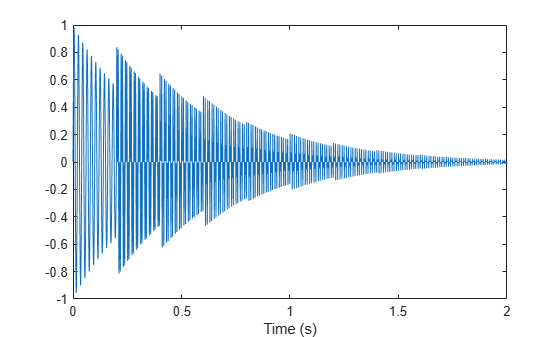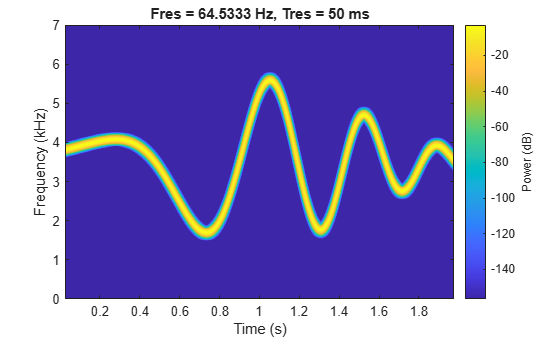Main Content

# instbw

Estimate instantaneous bandwidth

## Syntax

``ibw = instbw(x,fs)``
``ibw = instbw(x,t)``
``ibw = instbw(xt)``
``ibw = instbw(tfd,fd,td)``
``ibw = instbw(___,Name,Value)``
``[ibw,t] = instbw(___)``
``instbw(___)``

## Description

example

````ibw = instbw(x,fs)` estimates the instantaneous bandwidth of a signal, `x`, sampled at a rate `fs`. If `x` is a matrix, then the function estimates the instantaneous bandwidth independently for each column and returns the result in the corresponding column of `ibw`.```

example

````ibw = instbw(x,t)` estimates the instantaneous bandwidth of `x` sampled at the time values stored in `t`.```

example

````ibw = instbw(xt)` estimates the instantaneous bandwidth of a signal stored in the MATLAB® timetable `xt`. The function treats all variables in the timetable and all columns inside each variable independently.```

example

````ibw = instbw(tfd,fd,td)` estimates the instantaneous bandwidth of the signal whose time-frequency distribution, `tfd`, is sampled at the bandwidth values stored in `fd` and the time values stored in `td`.```
````ibw = instbw(___,Name,Value)` specifies additional options for any of the previous syntaxes using name-value arguments. You can specify the scale factor or the frequency limits used in the computation. For example, `'FrequencyLimits',[10 20]` computes the instantaneous bandwidth of the input in the range from 10 Hz to 20 Hz.```

example

````[ibw,t] = instbw(___)` also returns `t`, a vector of sample times corresponding to `ibw`.```

example

````instbw(___)` with no output arguments plots the estimated instantaneous bandwidth.```

## Examples

collapse all

Generate a signal sampled at 600 Hz for 2 seconds. The signal consists of a chirp with sinusoidally varying frequency content.

```fs = 6e2; x = vco(sin(2*pi*(0:1/fs:2)),[0.1 0.4]*fs,fs);```

Compute the spectrogram of the signal and display it as a waterfall plot.

```[p,f,t] = pspectrum(x,fs,'spectrogram'); waterfall(f,t,p') ax = gca; ax.XDir = 'reverse'; view(30,45)```Estimate and plot the instantaneous bandwidth of the signal.

```instbw(x,fs) ylim([0 50])```Generate a signal sampled at 2 kHz for 2 seconds. The signal consists of a superposition of exponentially damped sinusoids of increasing frequency that are added at regular intervals. Plot the signal.

```fs = 2000; t = 0:1/fs:2-1/fs; frq = [50:100:950]'; amp = (t > 4*(frq-frq(1))/fs); x = sum(amp.*sin(2*pi*t.*frq).*exp(-3*t)); % To hear, type sound(x,fs) plot(t,x) xlabel('Time (s)')```Compute and display the instantaneous bandwidth of the signal.

```[bw,bt] = instbw(x,t); plot(bt,bw) xlabel('Time (s)') ylabel('Bandwidth (Hz)')```Compute the spectrogram of the signal. Specify a time resolution of 100 milliseconds and 0 overlap between adjoining segments. Use the spectrogram to estimate the instantaneous frequency of the signal.

```[p,ff,tt] = pspectrum(x,t,'spectrogram','TimeResolution',0.1,'OverlapPercent',0); instfreq(p,ff,tt)```Use the spectrogram to compute the instantaneous bandwidth.

`instbw(p,ff,tt)`Generate a signal sampled at 14 kHz for 2 seconds. The frequency of the signal varies as a chirp modulated by a Gaussian. Save the signal as a MATLAB® timetable.

```fs = 14000; t = (0:1/fs:2)'; s = vco(chirp(t+.1,0,t(end),3).*exp(-2*(t-1).^2),[0.1 0.4]*fs,fs); sx = timetable(s,'SampleRate',fs);```

Compute the spectrogram of the signal. Specify a leakage of 0.2, a time resolution of 50 milliseconds, and 99% of overlap between adjoining segments. Display the spectrogram.

```opts = {'spectrogram','Leakage',0.2,'TimeResolution',0.05,'OverlapPercent',99}; [p,ff,tt] = pspectrum(sx,opts{:}); pspectrum(sx,opts{:})```Estimate and display the instantaneous bandwidth of the signal.

`instbw(p,ff,tt)`## Input Arguments

collapse all

Input signal, specified as a vector or matrix. If `x` is a vector, then `instbw` treats it as a single channel. If `x` is a matrix, then `instbw` computes the instantaneous bandwidth independently for each column and returns the result in the corresponding column of `ibw`.

Example: `sin(2*pi*(0:127)/16)+randn(1,128)/100` specifies a noisy sinusoid.

Example: `[2 1].*sin(2*pi*(0:127)'./[16 64])` specifies a two-channel sinusoid.

Data Types: `single` | `double`
Complex Number Support: Yes

Sample rate, specified as a positive scalar. The sample rate is the number of samples per unit time. If the unit of time is seconds, then the sample rate is in Hz.

Data Types: `single` | `double`

Sample times, specified as a real vector, a `duration` scalar, a `duration` array, or a `datetime` array.

• `duration` scalar — The time interval between consecutive samples of `x`.

• Real vector, `duration` array, or `datetime` array — The time instant corresponding to each element of `x`.

Example: `seconds(1)` specifies a 1-second lapse between consecutive measurements of a signal.

Example: `seconds(0:8)` specifies that a signal is sampled at 1 Hz for 8 seconds.

Data Types: `single` | `double` | `duration` | `datetime`

Input timetable. `xt` must contain increasing, finite row times.

If a timetable has missing or duplicate time points, you can fix it using the tips in Clean Timetable with Missing, Duplicate, or Nonuniform Times.

Example: `timetable(seconds(0:4)',randn(5,1))` specifies a random process sampled at 1 Hz for 4 seconds.

Example: `timetable(seconds(0:4)',randn(5,3),randn(5,4))` contains a three-channel random process and a four-channel random process, both sampled at 1 Hz for 4 seconds.

Data Types: `single` | `double`
Complex Number Support: Yes

Time-frequency distribution, specified as a matrix sampled at the frequencies stored in `fd` and the time values stored in `td`. This input argument is supported only when `'Method'` is set to `'tfmoment'`.

Example: `[p,f,t] = pspectrum(sin(2*pi*(0:511)/4),4,'spectrogram')` specifies the time-frequency distribution of a 1 Hz sinusoid sampled at 4 Hz for 128 seconds, and also the frequencies and times at which it is computed.

Data Types: `single` | `double`

Frequency and time values for time-frequency distribution, specified as vectors. These input arguments are supported only when `'Method'` is set to `'tfmoment'`.

Example: `[p,f,t] = pspectrum(sin(2*pi*(0:511)/4),4,'spectrogram')` specifies the time-frequency distribution of a 1 Hz sinusoid sampled at 4 Hz for 128 seconds, and also the frequencies and times at which it is computed.

Data Types: `single` | `double`

### Name-Value Pair Arguments

Specify optional comma-separated pairs of `Name,Value` arguments. `Name` is the argument name and `Value` is the corresponding value. `Name` must appear inside quotes. You can specify several name and value pair arguments in any order as `Name1,Value1,...,NameN,ValueN`.

Example: `'FrequencyLimits',[25 50]` computes the instantaneous bandwidth of the input in the range from 25 Hz to 50 Hz.

Frequency range, specified as a two-element vector in Hz. If not specified, `'FrequencyLimits'` defaults to `[0 fs/2]` for real-valued signals and to `[-fs/2 fs/2]` for complex-valued signals.

Data Types: `single` | `double`

Scaling factor for spectral moment, specified as a real scalar.

Data Types: `single` | `double`

## Output Arguments

collapse all

Instantaneous bandwidth, returned as a vector, a matrix, or a timetable with the same dimensions as the input.

Times of bandwidth estimates, returned as a real vector, a `duration` array, or a `datetime` array.

## More About

collapse all

### Instantaneous Bandwidth

The instantaneous bandwidth of a nonstationary signal is a time-varying parameter that relates to the spread of the instantaneous frequency about its average at a given time instant , .

`instbw` estimates the instantaneous bandwidth as the square-root of the second conditional spectral moment of the time-frequency distribution of the input signal. The function:

1. Computes the spectrogram power spectrum P(t,f) of the input using the `pspectrum` function and uses the spectrum as a time-frequency distribution.

2. Estimates the instantaneous bandwidth using

`${\sigma }_{f}{}^{2}\left(t\right)=\frac{\underset{0}{\overset{\infty }{\int }}{\left(f-{f}_{\text{inst}}\left(t\right)\right)}^{2}\text{\hspace{0.17em}}P\left(t,f\right)\text{\hspace{0.17em}}df}{\underset{0}{\overset{\infty }{\int }}P\left(t,f\right)\text{\hspace{0.17em}}df},$`

where ${f}_{\text{inst}}\left(t\right)$ is the instantaneous frequency returned by `instfreq` and estimated by

`${f}_{\text{inst}}\left(t\right)=\frac{{\int }_{0}^{\infty }f\text{\hspace{0.17em}}P\left(t,f\right)\text{\hspace{0.17em}}df}{{\int }_{0}^{\infty }P\left(t,f\right)\text{\hspace{0.17em}}df}.$`

 Boashash, B. “Estimating and Interpreting the Instantaneous Frequency of a Signal. I. Fundamentals.” Proceedings of the IEEE® 80, no. 4 (April 1992): 520–38. https://doi.org/10.1109/5.135376.

 Boashash, B. "Estimating and Interpreting The Instantaneous Frequency of a Signal. II. Algorithms and Applications." Proceedings of the IEEE 80, no. 4 (May 1992): 540–568. https://doi.org/10.1109/5.135378.

## Support

#### Deep Learning for Signal Processing with MATLAB

Download white paper